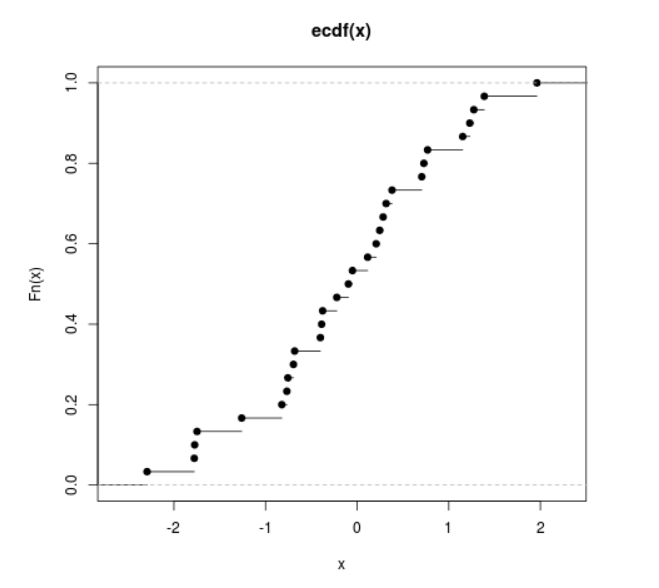# Compute the Value of Empirical Cumulative Distribution Function in R Programming – ecdf() Function

`ecdf()` function in R Language is used to compute and plot the value of Empirical Cumulative Distribution Function of a numeric vector.

Syntax: ecdf(x)

Parameters:
x: Numeric Vector

Example 1:

 `# R Program to compute the value of ` `# Empirical Cumulative Distribution Function ` ` `  `# Creating a Numeric Vector ` `x <``-` `seq(``1``, ``50``, by ``=` `2``) ` ` `  `# Calling ecdf() Function ` `y <``-` `ecdf(x) ` `y `

Output:

```Empirical CDF
Call: ecdf(x)
x[1:25] =      1,      3,      5,  ...,     47,     49
```

Example 2:

 `# R Program to compute the value of ` `# Empirical Cumulative Distribution Function ` ` `  `# Creating a random vector ` `x <``-` `rnorm(``30``) ` ` `  `# Calling ecdf() Function ` `y <``-` `ecdf(x) ` ` `  `# Plot a graph ` `plot(y) `

Output:My Personal Notes arrow_drop_upCheck out this Author's contributed articles.

If you like GeeksforGeeks and would like to contribute, you can also write an article using contribute.geeksforgeeks.org or mail your article to contribute@geeksforgeeks.org. See your article appearing on the GeeksforGeeks main page and help other Geeks.

Please Improve this article if you find anything incorrect by clicking on the "Improve Article" button below.

Article Tags :

Be the First to upvote.

Please write to us at contribute@geeksforgeeks.org to report any issue with the above content.